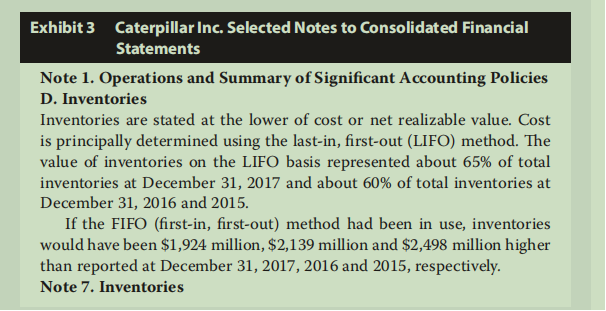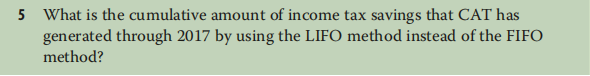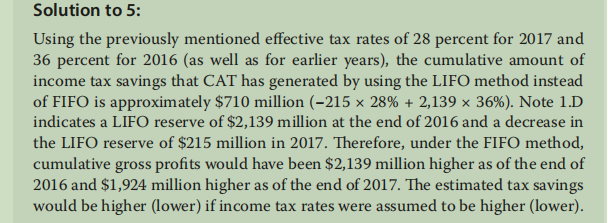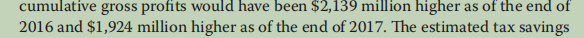# A question from the textbook

This is a sample question from p325 of the textbook:I thought the tax savings should be:
2139x36% + 1924x 28%
as under the FIFO method,Why the change in LIFO reserve of 215 was used in the answer?
Doesn’t the question ask about the total tax savings under LIFO, which is the tax savings in 2016 and 2017 in total?

Thank you very much for any insight!

2139 and 1924 are cumulative amounts. Thus, you calculate the 2016 amount using that years’ tax rate, and then the change from 2016 to 2017 using the 2017 tax rate. Because they are cumulative amounts, the 2017 amount is simply the difference between the 2016 and 2017 cumulative amounts.